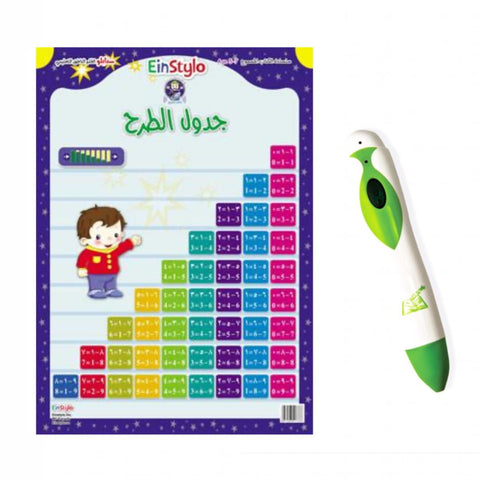## EinStylo - Subtraction Table (5-7 years) - Poster

• \$ 72.99
• Save \$ -56

Subtraction can be defined as a mathematical process that can be expressed by expressing the process of removing a certain number of things on the ground from a group that includes a greater number of them, thus resulting in getting fewer things in that group.

The subtraction operation in arithmetic is one of the four arithmetic operations, and it is the opposite of addition, and it is denoted by the sign of the minus.

Therefore, the Stoll Group offers a poster for the simple infraction operations for children as it explains the mathematical subtraction operations easily and simply while appropriate for the child's age.

The subtraction poster provides the numbers from 1 to 9, which are simple and easy arithmetic operations for the child in this age group presented in a fun way that helps in developing skills Cognitive and cognitive of the child

Features

• Subtraction Table (5-7 years) Simplified explanation of the mathematical subtraction from 1 to 9.
• Subtraction Table (5-7 years) Available in both Arabic and English.
• Subtraction Table (5-7 years) it develops the child's cognitive and cognitive skills.
• Subtraction Table (5-7 years) Suitable for this age group (5-7).
• You can get speaking ben to facilitate the educational process, as this pen acts as a teacher, so it is easy for children and parents to use.

We Also Recommend